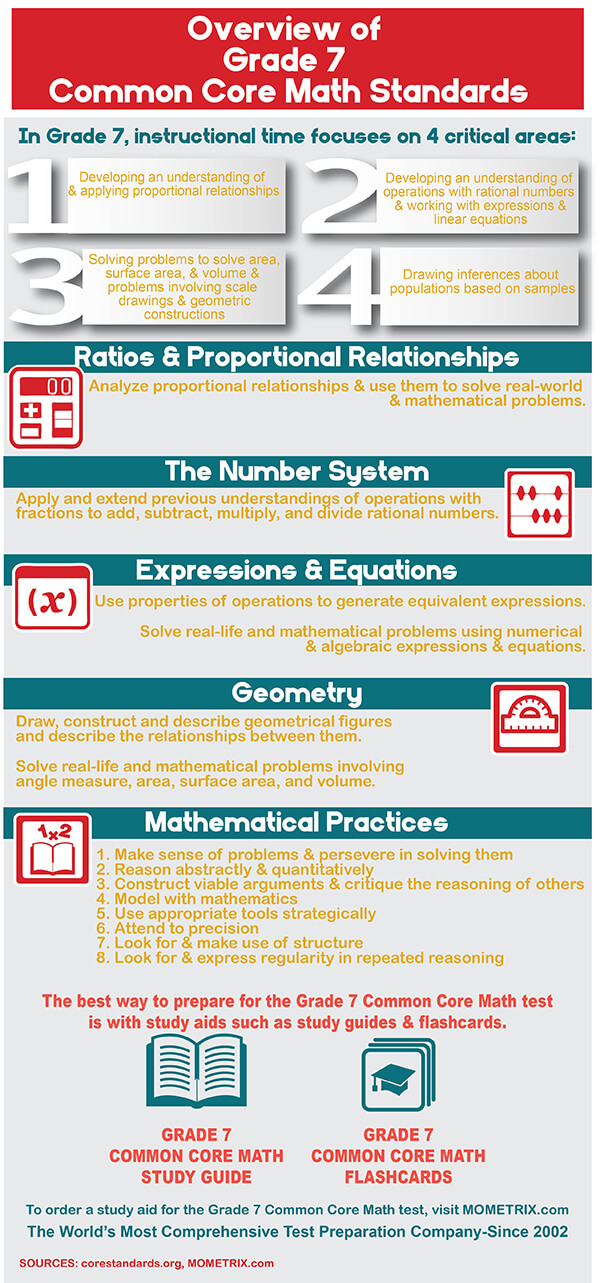# Mathematics Grade 7 Practice Questions

##### 1. Examine the figure below.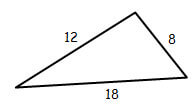1. 19
2. 32
3. 44
4. 76
##### 2. Examine the figures below.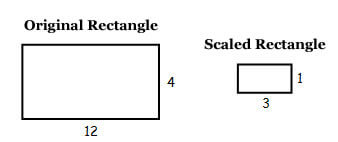1. ¼
2. 1/3
3. 3
4. 4
##### 3. Which of the following sets of angles CANNOT be used to construct a triangle?
1. Three acute angles
2. Two acute angles and one right angle
3. Two acute angles and one obtuse angle
4. Two right angles and one acute angle
##### 4. If the right rectangular prism shown below was horizontally sliced, which of the following shapes would BEST describe the resulting two-dimensional shape?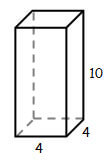1. Parallelogram
2. Rectangle
3. Rhombus
4. Square
1. 15.7 ft2
2. 31.4 ft2
3. 78.5 ft2
4. 314 ft2
1. 9.42 ft
2. 18.8 ft
3. 28.3 ft
4. 113 ft
##### 7. Examine the figure below.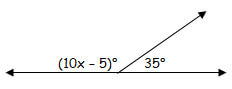1. 4
2. 6
3. 15
4. 21
##### 8. What is the measure of ?DBC?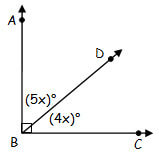1. 10°
2. 20°
3. 40°
4. 50°
##### 9. Jason is building a desk for his bedroom. He wants the desk to fit in the corner of his room and decides to go with a trapezoid design. If Jason uses the dimensions shown in the picture below, how many square feet of plywood will he need to build the desk top?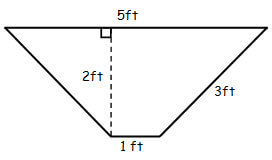1. 5 ft2
2. 6 ft2
3. 9 ft2
4. 12 ft2
##### 10. Natalie recently bought a jewelry box, but she wants to re-cover the box with a more colorful fabric. If the jewelry box is a cube with sides 8 cm long, how much fabric will Natalie need in order to cover all sides of the box?
1. 64 cm2
2. 192 cm2
3. 384 cm2
4. 512 cm2

1. D: Since the original triangle is dilated by a scale factor of 2, the length of each side will be multiplied by 2 to get the side lengths of the new triangle. Therefore, 8×2=16, 12×2=24, and 18×2=36. Then, 16+24+36=76. Answer A results if each of the side lengths was incorrectly divided by 2. Answer B results if 2 was incorrectly subtracted from each of the side lengths. Answer C results if 2 was incorrectly added to the length of each side.

2. A: To determine the scale factor, take the side lengths of the scaled rectangle and divide them by the corresponding sides of the original rectangle: 1/4=1/4 and 3/12=1/4. Therefore, the scale factor used to scale the rectangle is 1/4. Answer B results if the scale factor was incorrectly determined by dividing the sides within each rectangle in the order of 4/12=1/3and 1/3=1/3. Answer C results if the scale factor was incorrectly determined by dividing the sides within each rectangle in the order of 12/4=3 and 3/1=3. Answer D results if the scale factor was incorrectly set up to have the original side lengths divided by the values of the scaled side lengths: 12/3=4 and 4/1=4.

3. D: In order to construct a triangle, the three angles must sum to 180°. In Answer D, the two right angles by themselves would sum to180°, so adding an acute angle to that value would make the sum greater than 180°. Therefore, a triangle cannot be constructed with two right angles. Answers A, B, and C are all possible angle options for constructing a triangle.

4. D: Horizontally slicing the right rectangular prism would create slices that are parallel to the base of the prism. Since the base of the prism is a square with sides of length 4, each of the horizontal slices would also be in the shape of a square. Answers A and C are the shapes that the base appears to be if one does not take into account the drawing of the 3-D image and that the prism is a right rectangular prism. Answer B is the resulting two-dimensional shape if the prism was vertically sliced.

5. C: The formula for the area of a circle is A=pr2. Since the diameter of the garden is 10 ft, the radius of the garden is 5 ft. Therefore, A=3.14(5 “ft” )2=78.5″ft” 2. Answer A is the result if the radius was not squared, i.e. ?r. Answer B is the result if the circumference of the garden was calculated. Answer D is the result of using the diameter in the area formula.

6. B: The distance around each tire is the circumference of the circle: C=2pr=2(3.14)(3 “ft” )=18.84 “ft” . Answer A is the result if the radius was not doubled. Answer C is the area inside of the tire. Answer D is the result if the diameter was used in the area formula.

7. C: The two angles shown in the figure are supplementary. In order to solve for x, the following equation must be used: 10x-5+35=180. This equation simplifies as: 10x+30=180. After subtracting 30 from both sides, the equation becomes 10x=150. Finally, after dividing both sides by 10, the solution is x=15. Answer A results from treating the angles as congruent and setting up the equation as 10x-5=35. Answer B results from treating the angles as complementary and setting up the equation as 10x-5+35=90. Answer D results if the equation was correctly set up as 10x+30=180, but then 30 was incorrectly added to both sides to get 10x=210.

8. C: Before we can find measure of ?DBC, we first need to solve for x. Since ?ABD and ?DBC are complementary, we need to use the equation m ?ABD + m ?DBC = 90. Substituting for the values of m ?ABD and m ?DBC, the equation becomes 5x+4x=90. This equation simplifies as 9x=90. Finally, after dividing both sides of the equation by 9, the result isx=10. To find the measure ?DBC, we substitute x=10 into (4x)° to get (4(10))°=40°. Answer A is the value of x. Answer B is the value of x if the angles were treated as supplementary and set up as 5x+4x=180. Answer D is the measure of ?ABD.

9. B: The formula for the area of a trapezoid is A=1/2 h(b_1+b_2), where h is the height, and b1 & b2 are lengths of the two bases. Therefore, A=1/2 (2 “ft” )(5 “ft” +1 “ft” )=1/2 (2 “ft” )(6 “ft” )=6 ?”ft” ?^2. In Answer A, only the 5 ft base was used in the calculation. In Answer C, the 3 ft value was used for the height. In Answer D, the 1/2 was left out of the formula, so the area was incorrectly calculated as A=h(b_1+b_2).

10. C: The amount of fabric needed to cover the jewelry box is equal to the surface area of the box. The formula for the surface area of a cube is SA=62, where s is the length of one side. Therefore, the surface area of the jewelry box is SA=6(8 cm )2= 6(64cm)2 =384 cm 2. In Answer A, the area of only one side of the box was calculated. In Answer B, the perimeter of one side of the box was multiplied by 6. In Answer D, the volume of the box was calculated.